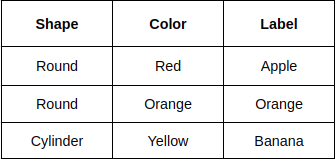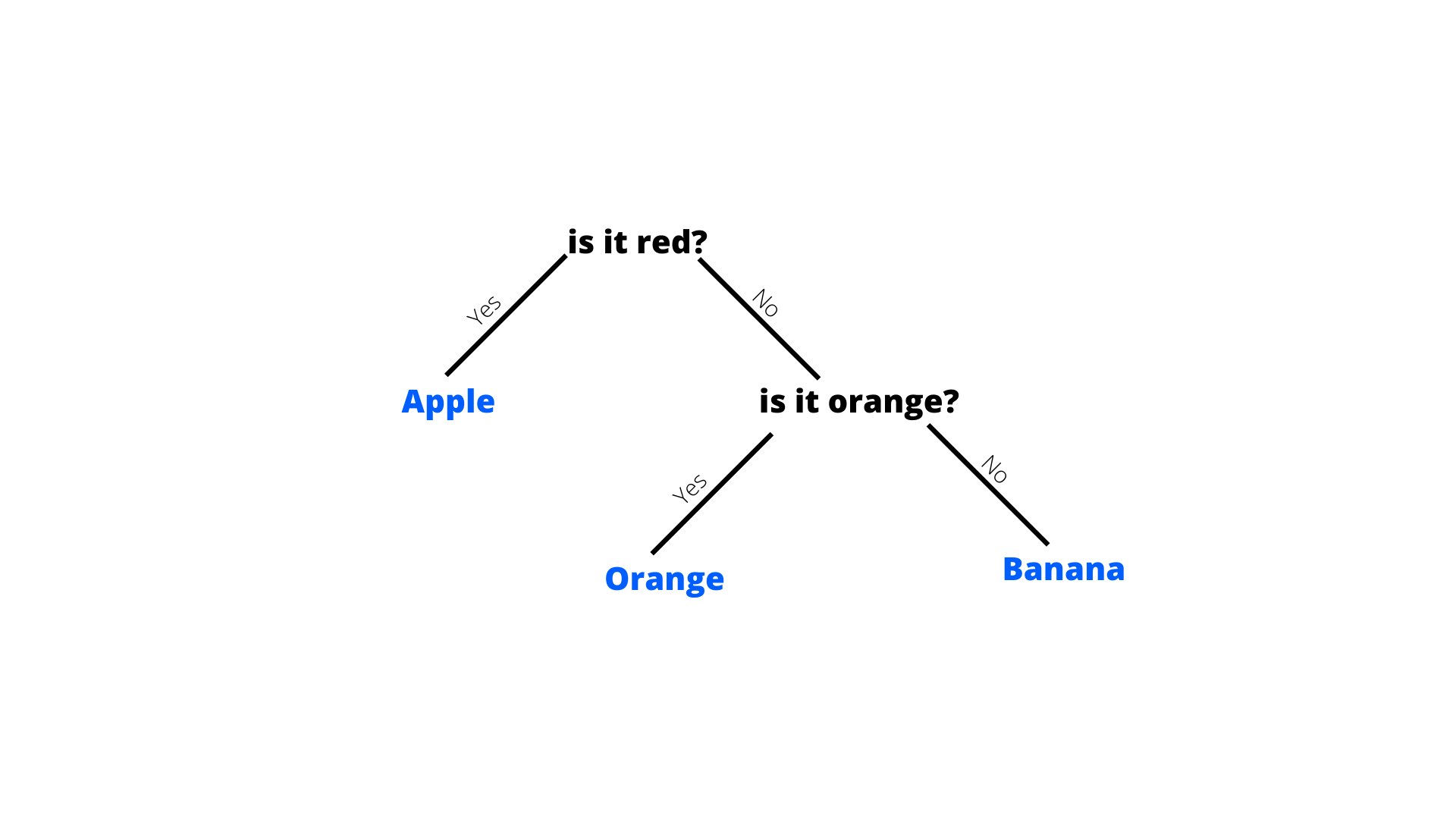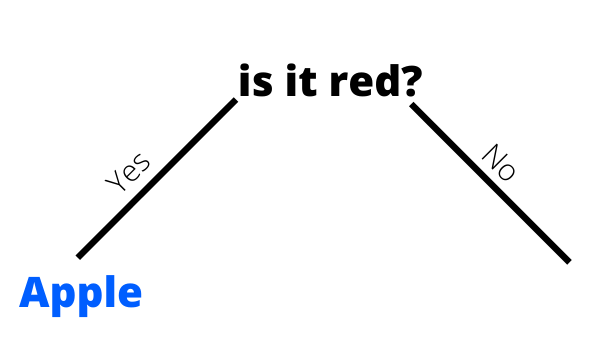Idiomatic Programmers 2021-08-26T18:51:43.821Z https://github.com/nuxt-community/feed-module A place where you can get high quality tutorials, text based courses and tips related to Python, Django, DRF, Machine Learning and Computer Science <![CDATA[The Mathematics in Decision Trees]]> https://idiomaticprogrammers.com/post/the-mathematics-in-decision-trees/ 2019-12-20T21:04:56.000ZDecision Trees is a way of representing the relationship between different attributes in a given dataset using Binary Tree data structure such that this representation can be used to do future predictions or classification.

An abstract way of understanding the Decision Tree algorithm is that it asks a series of questions based on the dataset to construct a binary tree representation. Let’s say we have to classify an image of Apple, Orange, and Banana and we have the following dataset.So based on these data, the algorithm can create a Decision Tree like this.In the beginning, the entire dataset is kept at the root node of the tree and then with each iteration, a subset of the root dataset is split and added as it’s a child.

The major challenge in the Decision Tree algorithm is to identify which attribute must be selected to split from the parent node to add as the child node (also known as “ask a question”) and thus can maximise the prediction accuracy. There are two major metrics that help us to achieve this task.

1. Information Gain
2. Gini Index

## Information Gain

Whenever the algorithm has to split the dataset into a subset of that dataset the entropy of the dataset changes. Information Gain is the measure of this change in entropy.

Suppose, S is a set of instances, A is an attribute

$Gain(S,A) = Entropy(S) - Entropy(A)$

### Entropy

Entropy or Information Entropy is the measure of randomness in a given piece of information. According to Wikipedia, Information entropy is the average rate at which information is produced by a stochastic source of data.

Entropy is defined as the negative mean of logarithmic probabilities.

$Entropy(x) = -(x)log_2P(x) \forall x \in X$

In terms of a Decision Tree, the probability P will be the probability of occurrence of an element in a subset of the dataset. Let’s take an example of the attribute “Shape” in Table 1.

The “Shape” has 2 instances of “Round” and out of them, 1 corresponds to Yes (Apple) and 1 corresponds to No (Not Apple). Also, “Shape” has 1 instance of “Cylinder” which corresponds to not Apple. Given this information, the Entropy of Age will be:

$S*{shape}^{round} = -\frac{1}{2}log_2(\frac{1}{2})-\frac{1}{2}log_2(\frac{1}{2})$
$S*{shape}^{round} = -(0.5\times-1)-(0.5\times-1)=1$
$S*{shape}^{cylinder} = -\frac{0}{1}log_2(\frac{0}{1})-\frac{1}{1}log_2(\frac{1}{1})$
$S*{shape}^{round} = -(0\times1)-(1\times-0)=0$
$S_{shape} = \frac{2}{3}\times1+\frac{1}{3}\times0 = 0.67$

When we implement Decision Tree using Information Gain metric, for every iteration we calculate the Information Gain for each attribute in a root node. Let’s see how the decision tree in Fig. 1 is constructed.

Our example dataset consists of 2 feature attribute (shape and colour) and 3 classes (apple, orange, and banana). So in the first iteration, we will classify one of the classes let’s say Apple

Iteration 1: At the first iteration, we assume that the Entropy of the Root Node is 1 and we assign the entire dataset to it. Then we calculate the Information Gain for each Attribute in our dataset (Shape and Color in this case). We already calculated the entropy for shape, now let's calculate the entropy for colour.

$S_{color}^{red} = -\frac{1}{1} \times log_2(\frac{1}{1}) -\frac{0}{1} \times log_2(\frac{0}{1})$
$S_{color}^{red} = -1 \times 0 - 0 \times 1 = 0$
$S_{color}^{orange} = -\frac{0}{1} \times log_2(\frac{0}{1}) -\frac{1}{1} \times log_2(\frac{1}{1})$
$S_{color}^{red} = -0 \times 1 - 1 \times 0 = 0$
$S_{color}^{yellow} = -\frac{0}{1} \times log_2(\frac{0}{1}) -\frac{1}{1} \times log_2(\frac{1}{1})$
$S_{color}^{yellow} = -0 \times 1 - 1 \times 0 = 0$
$S_{color} = -\frac{1}{3} \times 0 -\frac{1}{3} \times 0 -\frac{1}{3} \times 0$

We then calculate Information Gain for Shape and Colour.

$Gain_{shape} = 1 - 0.67 = 0.33$
$Gain_{color} = 1 - 0 = 1$

We will select the attribute that has the maximum Gain, in this case, Colour. So at this point, our tree looks like this:Iteration 2 Since we found a leaf node (class) in the first iteration, we can move forward to represent the next class, let’s say orange. Otherwise, we would have continued with Apple until we find a leaf node (which can be identified by an Information Gain value of 1).

Now we again have to calculate Entropy and Information Gain with respect to class Orange.

$S*{shape}^{round} = -\frac{1}{2}log_2(\frac{1}{2})-\frac{1}{2}log_2(\frac{1}{2})$
$S*{shape}^{round} = -(0.5\times-1)-(0.5\times-1)=1$
$S*{shape}^{cylinder} = -\frac{0}{1}log_2(\frac{0}{1})-\frac{1}{1}log_2(\frac{1}{1})$
$S*{shape}^{round} = -(0\times1)-(1\times-0)=0$
$S_{shape} = \frac{2}{3}\times1+\frac{1}{3}\times0 = 0.67$
$S_{color}^{red} = -\frac{1}{1} \times log_2(\frac{1}{1}) -\frac{0}{1} \times log_2(\frac{0}{1})$
$S_{color}^{red} = -1 \times 0 - 0 \times 1 = 0$
$S_{color}^{orange} = -\frac{0}{1} \times log_2(\frac{0}{1}) -\frac{1}{1} \times log_2(\frac{1}{1})$
$S_{color}^{red} = -0 \times 1 - 1 \times 0 = 0$
$S_{color}^{yellow} = -\frac{0}{1} \times log_2(\frac{0}{1}) -\frac{1}{1} \times log_2(\frac{1}{1})$
$S_{color}^{yellow} = -0 \times 1 - 1 \times 0 = 0$
$S_{color} = -\frac{1}{3} \times 0 -\frac{1}{3} \times 0 -\frac{1}{3} \times 0$
$Gain_{shape} = 1 - 0.67 = 0.33$
$Gain_{color} = 1 - 0 = 1$

Coincidentally, the calculation of Apple and Oranges and exactly the same. We have to select the attribute with maximum Information gain. And since this is a leaf node and there is only one class left to classify, we will directly classify the Banana based on the question for Orange. Something like this:That’s it for this blog, if you find any mistake then feel free to comment below.

]]>